mscroggs.co.uk
mscroggs.co.uksubscribe

# Blog

2019-07-03
This year's Big Internet Math-Off is now underway with 15 completely new contestants (plus one returning contender). As I'm not the returning contestant, I haven't been spending my time preparing my pitches. Instead, I've spent my time making an unofficial Big Internet Math-Off sticker book.
To complete the sticker book, you will need to collect 162 different stickers. Every day, you will be given a pack of 5 stickers; there are also some bonus packs available if you can find them (Hint: keep reading).

### How many stickers will I need?

Using the same method as I did for last year's World Cup sticker book, you can work out that the expected number of stickers needed to finish the sticker book:
If you have already stuck $$n$$ stickers into your album, then the probability that the next sticker you get is new is
$$\frac{162-n}{162}.$$
The probability that the second sticker you get is the next new sticker is
$$\mathbb{P}(\text{next sticker is not new})\times\mathbb{P}(\text{sticker after next is new})$$ $$=\frac{n}{162}\times\frac{162-n}{162}.$$
Following the same method, we can see that the probability that the $$i$$th sticker you buy is the next new sticker is
$$\left(\frac{n}{162}\right)^{i-1}\times\frac{162-n}{162}.$$
Using this, we can calculate the expected number of stickers you will need to buy until you find a new one:
$$\sum_{i=1}^{\infty}i \left(\frac{162-n}{162}\right) \left(\frac{n}{162}\right)^{i-1} = \frac{162}{162-n}$$
Therefore, to get all 162 stickers, you should expect to buy
$$\sum_{n=0}^{161}\frac{162}{162-n} = 918 \text{ stickers}.$$
Using just your daily packs, it will take you until the end of the year to collect this many stickers. Of course, you'll only need to collect this many if you don't swap your duplicate stickers.

### How many stickers will I need if I swap?

To work out the expected number of stickers stickers you'd need if you swap, let's first think about two people who want to complete their stickerbooks together. If there are $$a$$ stickers that both collectors need and $$b$$ stickers that one collector has and the other one needs, then let $$E_{a,b}$$ be the expected number of stickers they need to finish their sticker books. The next sticker they get could be one of three things:
• A sticker they both need (with probability $$\frac{a}{162}$$);
• A sticker one of them needs (with probability $$\frac{b}{162}$$);
• A sticker they both have (with probability $$\frac{162-a-b}{162}$$).
Therefore, the expected number of stickers they need to complete their sticker books is
$$E_{a,b}=1+\frac{a}{162}E_{a-1,b+1}+\frac{b}{162}E_{a,b-1}+\frac{162-a-b}{162}E_{a,b}.$$
This can be rearranged to give
$$E_{a,b}= \frac{162}{a+b}+ \frac{a}{a+b}E_{a-1,b+1} +\frac{b}{a+b}E_{a,b-1}$$
We know that $E_{0,0}=0$ (as if $$a=0$$ and $$b=0$$, both collectors have already finished their sticker books). Using this and the formula above, we can work out that
$$E_{0,1}=162+E_{0,0}=162$$ $$E_{1,0}=162+E_{0,1}=324$$ $$E_{0,2}=\frac{162}2+E_{0,1}=243$$ $$E_{1,1}=\frac{162}2+\frac12E_{0,2}+\frac12E_{1,0}=364.5$$
... and so on until we find that $$E_{162,0}=1269$$, and so our collectors should expect to collect 634 stickers each to complete their sticker books.
For three people, we can work out that if there are $$a$$ stickers that all three need, $$b$$ stickers that two need, and $$c$$ stickers that one needs, then
$$E_{a,b,c} = \frac{162}{a+b+c}+ \frac{a}{a+b+c}E_{a-1,b+1,c} +\frac{b}{a+b+c}E_{a,b-1,c+1} +\frac{c}{a+b+c}E_{a,b,c-1}.$$
In the same way as for two people, we find that $$E_{162,0,0}=1572$$, and so our collectors should expect to collect 524 stickers each to complete their sticker books.
Doing the same thing for four people gives an expected 463 stickers required each.
After four people, however, the Python code I wrote to do these calculations takes too long to run, so instead I approximated the numbers by simulating 500 groups of $$n$$ people collecting stickers, and taking the average number of stickers they needed. The results are shown in the graph below.
The red dots are the expected values we calculated exactly, and the blue crosses are the simulated values. It looks like you'll need to collect at least 250 stickers to finish the album: in order to get this many before the end of the Math-Off, you'll need to find 20 bonus packs...
Of course, these are just the mean values and you could get lucky and need fewer stickers. The next graph shows box plots with the quartiles of the data from the simulations.
So if you're lucky, you could complete the album with fewer stickers or fewer friends.
As a thank you for reading to the end of this blog post, here's a link that will give you two bonus packs and help you on your way to the 250 expected stickers...

### Similar postsRunge's Phenomenon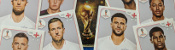World Cup stickers 2018, pt. 3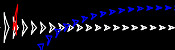Mathsteroids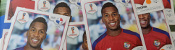World Cup stickers 2018, pt. 2

Comments in green were written by me. Comments in blue were not written by me.
Link to sticker book, in the first paragraph, does not work. It points to mathoffstickbook.com
Pat Ashforth
minor typo for the 2 collector case

> and so our collectors should expect to collect 364 stickers

should be 634.

Allowed HTML tags: <br> <a> <small> <b> <i> <s> <sup> <sub> <u> <spoiler> <ul> <ol> <li>
To prove you are not a spam bot, please type "orez" backwards in the box below (case sensitive):
2019-03-26
I originally wrote this post for The Aperiodical.
A few months ago, Adam Townsend went to lunch and had a conversation. I wasn't there, but I imagine the conversation went something like this:Smitha: Hello.
Smitha: You know how the \hat command in LaTeΧ puts a caret above a letter?... Well I was thinking it would be funny if someone made a package that made the \hat command put a picture of an actual hat on the symbol instead?
Adam: (After a few hours of laughter.) I'll see what my flatmate is up to this weekend...
Jeff: What on Earth are you two talking about?!As anyone who has been anywhere near maths at a university in the last ∞ years will be able to tell you, LaTeΧ is a piece of maths typesetting software. It's a bit like a version of Word that runs in terminal and makes PDFs with really pretty equations.
By default, LaTeΧ can't do very much, but features can easily added by importing packages: importing the graphicsx package allows you to put images in your PDF; importing geometry allows you to easily change the page margins; and importing realhats makes the \hat command put real hats above symbols.

### Changing the behaviour of \hat

By default, the LaTeΧ command \hat puts a pointy "hat" above a symbol:
After Adam's conversation, we had a go at redefining the \hat command by putting the following at the top of our LaTeΧ file.
LaTeΧ
\renewcommand{\hat}{
% We put our new definition here
}

After a fair amount of fiddling with the code, we eventually got it to produce the following result: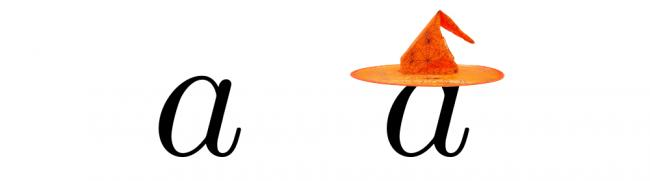a (left) and \hat{a} (right) while using the realhats package
We were now ready to put our code into a package so others could use it.

### How to write a package

A LaTeΧ package is made up of:
• a sty file, containing a collection of commands like the one we wrote above;
• a PDF of documentation showing users how to use your package;
It's quite common to make the first two of these by making a dtx file and an ins file. And no, we have no idea either why these are the file extensions used or why this is supposedly simpler than making a sty file and a PDF.
The ins file says which bits of the dtx should be used to make up the sty file. Our ins file looks like this:
LaTeΧ
\input{docstrip.tex}
\keepsilent
\usedir{tex/latex/realhats}
\preamble
\endpreamble
\generate{
\file{realhats.sty}{\from{realhats.dtx}{realhats}}
}
\endbatchfile
The most important command in this file is \generate: this says that that the file realhats.sty should be made from the file realhats.dtx taking all the lines that are marked as part of realhats. The following is part of our dtx file:
LaTeΧ
%\lstinline{realhats} is a package for \LaTeX{} that makes the \lstinline{\hat}
%command put real hats on symbols.
%For example, the input \lstinline@\hat{a}=\hat{b}@ will produce the output:
%$\hat{a}=\hat{b}$
%To make a vector with a hat, the input \lstinline@\hat{\mathbf{a}}@ produces:
%$\hat{\mathbf{a}}$
%
%\iffalse
%<*documentation>
\documentclass{article}
\usepackage{realhats}
\usepackage{doc}
\usepackage{listings}
\title{realhats}
\begin{document}
\maketitle
\DocInput{realhats.dtx}
\end{document}
%</documentation>
%\fi

%\iffalse
%<*realhats>
\NeedsTeXFormat{LaTeX2e}
\ProvidesPackage{realhats}[2019/02/02 realhats]

\RequirePackage{amsmath}
\RequirePackage{graphicx}
\RequirePackage{ifthen}
\renewcommand{\hat}{
% We put our new definition here
}
%</realhats>
%\fi
The lines near the end between <*realhats> and </realhats> will be included in the sty file, as they are marked at part of realhats.
The rest of this file will make the PDF documentation when the dtx file is compiled. The command \DocInput tells LaTeΧ to include the dtx again, but with the %s that make lines into comments removed. In this way all the comments that describe the functionality will end up in the PDF. The lines that define the package will not be included in the PDF as they are between \iffalse and \fi.
Writing both the commands and the documentation in the same file like this means that the resulting file is quite a mess, and really quite ugly. But this is apparently the standard way of writing LaTeΧ packages, so rest assured that it's not just our code that ugly and confusing.

### What to do with your package

Once you've written a package, you'll want to get it out there for other people to use. After all, what's the point of being able to put real hats on top of symbols if the whole world can't do the same?
First, we put the source code of our package on GitHub, so that Adam and I had an easy way to both work on the same code. This also allows other LaTeΧ lovers to see the source and contribute to it, although none have chosen to add anything yet.
Next, we submitted our package to CTAN, the Comprehensive TeΧ Archive Network. CTAN is an archive of thousands of LaTeΧ packages, and putting realhats there gives LaTeΧ users everywhere easy access to real hats. Within days of being added to CTAN, realhats was added (with no work by us) to MikTeX and TeX Live to allow anyone using these LaTeΧ distributions to seemlessly install it as soon as it is needed.
We figured that the packaged needed a website too, so we made one. We also figured that the website should look as horrid as possible.

### How to use realhats

So if you want to end fake hats and put real hats on top of your symbols, you can simply write \usepackage{realhats} at the top of your LaTeΧ file.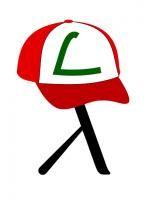realhats: gotta put them all in academic papers

### Similar posts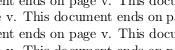A non-converging LaTeX documentBig Internet Math-Off stickers 2019Runge's PhenomenonMathsteroids

Comments in green were written by me. Comments in blue were not written by me.

Allowed HTML tags: <br> <a> <small> <b> <i> <s> <sup> <sub> <u> <spoiler> <ul> <ol> <li>
To prove you are not a spam bot, please type "emirp" backwards in the box below (case sensitive):
2018-09-13
This is a post I wrote for round 2 of The Aperiodical's Big Internet Math-Off 2018. As I went out in round 1 of the Big Math-Off, you got to read about the real projective plane instead of this.
Polynomials are very nice functions: they're easy to integrate and differentiate, it's quick to calculate their value at points, and they're generally friendly to deal with. Because of this, it can often be useful to find a polynomial that closely approximates a more complicated function.
Imagine a function defined for $$x$$ between -1 and 1. Pick $$n-1$$ points that lie on the function. There is a unique degree $$n$$ polynomial (a polynomial whose highest power of $$x$$ is $$x^n$$) that passes through these points. This polynomial is called an interpolating polynomial, and it sounds like it ought to be a pretty good approximation of the function.
So let's try taking points on a function at equally spaced values of $$x$$, and try to approximate the function:
$$f(x)=\frac1{1+25x^2}$$
Polynomial interpolations of $$\displaystyle f(x)=\frac1{1+25x^2}$$ using equally spaced points
I'm sure you'll agree that these approximations are pretty terrible, and they get worse as more points are added. The high error towards 1 and -1 is called Runge's phenomenon, and was discovered in 1901 by Carl David Tolmé Runge.
All hope of finding a good polynomial approximation is not lost, however: by choosing the points more carefully, it's possible to avoid Runge's phenomenon. Chebyshev points (named after Pafnuty Chebyshev) are defined by taking the $$x$$ co-ordinate of equally spaced points on a circle.
The following GIF shows interpolating polynomials of the same function as before using Chebyshev points.
Nice, we've found a polynomial that closely approximates the function... But I guess you're now wondering how well the Chebyshev interpolation will approximate other functions. To find out, let's try it out on the votes over time of my first round Big Internet Math-Off match.
The graphs below show the results of the match over time interpolated using 16 uniform points (left) and 16 Chebyshev points (right). You can see that the uniform interpolation is all over the place, but the Chebyshev interpolation is very close the the actual results.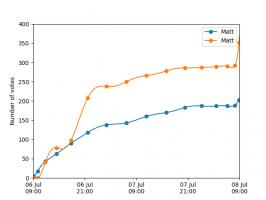Scroggs vs Parker, 6-8 July 2018, approximated using uniform points (left) and Chebyshev points (right)
But maybe you still want to see how good Chebyshev interpolation is for a function of your choice... To help you find out, I've written @RungeBot, a Twitter bot that can compare interpolations with equispaced and Chebyshev points. Just tweet it a function, and it'll show you how bad Runge's phenomenon is for that function, and how much better Chebysheb points are.
A list of constants and functions that RungeBot understands can be found here.

### Similar postsBig Internet Math-Off stickers 2019Mathsteroidsrealhats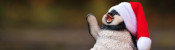Christmas (2019) is over

Comments in green were written by me. Comments in blue were not written by me.

Allowed HTML tags: <br> <a> <small> <b> <i> <s> <sup> <sub> <u> <spoiler> <ul> <ol> <li>
To prove you are not a spam bot, please type "w" then "i" then "d" then "t" then "h" in the box below (case sensitive):
2018-07-06
This is a post I wrote for round 1 of The Aperiodical's Big Internet Math-Off 2018, where Mathsteroids lost to MENACE.
A map projection is a way of representing the surface of a sphere, such as the Earth, on a flat surface. There is no way to represent all the features of a sphere on a flat surface, so if you want a map that shows a certain feature of the world, then you map will have to lose some other feature of the world in return.
To show you what different map projections do to a sphere, I have created a version of the game Asteroids on a sphere. I call it Mathsteroids. You can play it here, or follow the links below to play on specific projections.

### Mercator projection

The most commonly used map projection is the Mercator projection, invented by Gerardus Mercator in 1569. The Mercator projection preserves angles: if two straight lines meet at an angle $$\theta$$ on a sphere, then they will also meet at an angle $$\theta$$ on the map. Keeping the angles the same, however, will cause the straight lines to no longer appear straight on the map, and the size of the same object in two different place to be very different.
The angle preserving property means that lines on a constant bearing (eg 030° from North) will appear straight on the map. These constant bearing lines are not actually straight lines on the sphere, but when your ship is already being buffeted about by the wind, the waves, and the whims of drunken sailors, a reasonably straight line is the best you can expect.
The picture below shows what three ships travelling in straight lines on the sphere look like on a Mercator projection.
To fully experience the Mercator projection, you can play Mathsteroids in Mercator projection mode here. Try flying North to see your spaceship become huge and distorted.

### Gall–Peters projection

The Gall–Peters projection (named after James Gall and Arno Peters) is an area-preserving projection: objects on the map will have the same area as objects on the sphere, although the shape of the object on the projection can be very different to its shape on the sphere.
The picture below shows what three spaceships travelling in straight lines on a sphere look like on the Gall–Peters projection.
You can play Mathsteroids in Gall–Peters projection mode here. I find this one much harder to play than the Mercator projection, as the direction you're travelling changes in a strange way as you move.

### Azimuthal projection

An azimuthal projection makes a map on which the directions from the centre point to other points on the map are the same as the directions on the sphere. A map like this could be useful if, for example, you're a radio operator and need to quickly see which direction you should point your aerial to communicate with other points on the map.
The azimuthal projection I've used in Mathsteroids also preserves distances: the distance from the centre to the another points on the map is proportional to the actual distance on the sphere. This projection is used as the emblem of the UN.
The picture below shows three straight lines on this projection. You can play Mathsteroids in azimuthal mode here.### Craig retroazimuthal projection

A retroazimuthal projection makes a map on which the directions to the centre point from other points on the map are the same as the directions on the sphere. If you're thinking that this is the same as the azimuthal projection, then you're too used to doing geometry on flat surfaces: on a sphere, the sum of the angles in a triangle depends on the size of the triangle, so the directions from A to B and from B to A aren't as closely related as you would expect.
The Craig retroazimuthal projection was invented by James Ireland Craig in 1909. He used Mecca as his centre point to make a map that shows muslims across the world which direction they should face to pray.
The picture below shows what three spaceships travelling in a straight lines on a sphere looks like on this projection.
You can play Mathsteroids in Craig retroazimuthal mode here to explore the projection yourself. This is perhaps the hardest of all to play, as (a) two different parts of the sphere overlap on the map, and (b) the map is actually infinitely tall, so quite a bit of it is off the edge of the visible game area.

### Stereographic projection

The final projection I'd like to show you is the stereographic projection.
Imagine that a sphere is sitting on a 2D plane. Take a point on the sphere. Imagine a straight line through this point and the point at the top of the sphere. The point where this line meets the 2D plane is stereographic projection of the point on the sphere.
This projection (backwards) can be used to represent the every complex number as a point on a sphere: this is called the Riemann sphere.
To make Mathseteroids playable after this projection, I split the sphere into 2 hemisphere and projected each seperately to give two circles. You can play Mathsteroids in stereographic projection mode here. Three spaceships travelling in straight lines on this projection are shown below.
... and if you still don't like map projections, you can still enjoy playing Mathsteroids on an old fashioned torus. Or on a Klein bottle or the real projective plane. Don't forget to take a short break from playing to head over to The Aperiodical and vote (voting now closed).

### Similar posts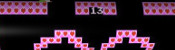Video game surfacesBig Internet Math-Off stickers 2019Runge's Phenomenon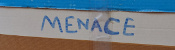Building MENACEs for other games

Comments in green were written by me. Comments in blue were not written by me.